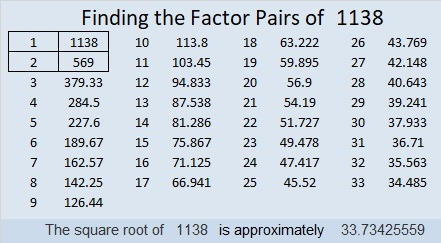# 1138 and Level 3

There are two common factors of 10 and 14, but only one of them will put only numbers from 1 to 12 in the first column. Do you know what that factor is? If you do, figure out where to put the factors of 22, 66, 15 and so forth to make this puzzle function like a multiplication table. Each number from 1 to 12 can only appear once in the first column and once in the top row. You can do this!Print the puzzles or type the solution in this excel file: 12 factors 1134-1147

Now I’d like to share some facts about the number 1138:

• 1138 is a composite number.
• Prime factorization: 1138 = 2 × 569
• The exponents in the prime factorization are 1 and 1. Adding one to each and multiplying we get (1 + 1)(1 + 1) = 2 × 2 = 4. Therefore 1138 has exactly 4 factors.
• Factors of 1138: 1, 2, 569, 1138
• Factor pairs: 1138 = 1 × 1138 or 2 × 569
• 1138 has no square factors that allow its square root to be simplified. √1138 ≈ 33.7342633² + 7² = 1138

1138 is the hypotenuse of a Pythagorean triple:
462-1040-1138 calculated from 2(33)(7), 33² – 7², 33² + 7²

This site uses Akismet to reduce spam. Learn how your comment data is processed.